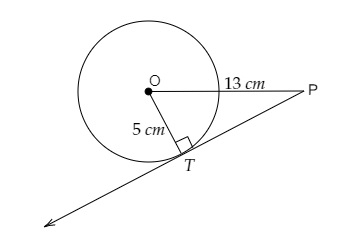# Find the length of a tangent drawn to a circle with radius 5 cm, from a point 13 cm from the centre of the circle.

Given:

Radius of the circle $=5\ cm$.

A point is 13 cm from the centre of the circle.

To do:

We have to find the length of the tangent.

Solution:

Let $O$ be the centre of the circle, $P$ be the point 13 cm from the centre of the circle and $PT$ be the tangent to the circle meeting it at $T$.$OT = 5\ cm$ and $OP = 13\ cm$

$PT$ is the tangent to the circle at $T$.

$OT\ perp\ PT$

In right angled triangle $OTP$,

By Pythagoras theorem,

$OP^2= OT^2+ TP^2$

$13^2 = 5^2 + TP^2$

$TP^2= 169 - 25$

$= 144$

$= 12^2$

Therefore,

$TP = 12\ cm$

The length of the tangent is 12 cm.

Updated on: 10-Oct-2022

51 Views